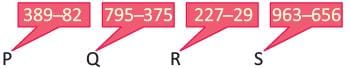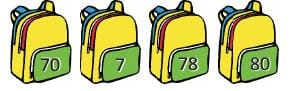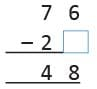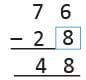Olympiad Test Level 2: Addition and Subtraction - 2

# Olympiad Test Level 2: Addition and Subtraction - 2

Test Description

## 10 Questions MCQ Test Math Olympiad for Class 2 | Olympiad Test Level 2: Addition and Subtraction - 2

Olympiad Test Level 2: Addition and Subtraction - 2 for Class 2 2022 is part of Math Olympiad for Class 2 preparation. The Olympiad Test Level 2: Addition and Subtraction - 2 questions and answers have been prepared according to the Class 2 exam syllabus.The Olympiad Test Level 2: Addition and Subtraction - 2 MCQs are made for Class 2 2022 Exam. Find important definitions, questions, notes, meanings, examples, exercises, MCQs and online tests for Olympiad Test Level 2: Addition and Subtraction - 2 below.
Solutions of Olympiad Test Level 2: Addition and Subtraction - 2 questions in English are available as part of our Math Olympiad for Class 2 for Class 2 & Olympiad Test Level 2: Addition and Subtraction - 2 solutions in Hindi for Math Olympiad for Class 2 course. Download more important topics, notes, lectures and mock test series for Class 2 Exam by signing up for free. Attempt Olympiad Test Level 2: Addition and Subtraction - 2 | 10 questions in 20 minutes | Mock test for Class 2 preparation | Free important questions MCQ to study Math Olympiad for Class 2 for Class 2 Exam | Download free PDF with solutions
 1 Crore+ students have signed up on EduRev. Have you?
Olympiad Test Level 2: Addition and Subtraction - 2 - Question 1

### If (+) means (-) and (-) means (+), then find the value of 18 - 16 + 12.

Detailed Solution for Olympiad Test Level 2: Addition and Subtraction - 2 - Question 1

Given 18 – 16 + 12
After Changing signs,
18 + 16 - 12 = 22

Olympiad Test Level 2: Addition and Subtraction - 2 - Question 2

### Which two sets of values are equal ?Detailed Solution for Olympiad Test Level 2: Addition and Subtraction - 2 - Question 2

P = 389 – 82 = 307
Q = 795 – 375 = 420
R = 227 – 29 = 198
S = 963 – 656 = 307
Hence P and S are equal

Olympiad Test Level 2: Addition and Subtraction - 2 - Question 3

### The sum of the numbers in the given bag is :Detailed Solution for Olympiad Test Level 2: Addition and Subtraction - 2 - Question 3

70 + 7 + 78 + 80 = 235

Olympiad Test Level 2: Addition and Subtraction - 2 - Question 4

On subtracting the sum of 47 and 16 from 125, we get :

Detailed Solution for Olympiad Test Level 2: Addition and Subtraction - 2 - Question 4

47 + 16 = 63
Now, 125 – 63 = 62

Olympiad Test Level 2: Addition and Subtraction - 2 - Question 5

Which numbers complete the pattern?
6 – 5 = ______
60 – 50 = ______

Detailed Solution for Olympiad Test Level 2: Addition and Subtraction - 2 - Question 5

6 – 5 = 1
60 – 50 = 10

Olympiad Test Level 2: Addition and Subtraction - 2 - Question 6

A book has 98 pages. Gauri has read 59 pages out of them. How many more pages does she has to read to finish the book ?

Detailed Solution for Olympiad Test Level 2: Addition and Subtraction - 2 - Question 6

Remaining pages = 98 – 59 = 39

Olympiad Test Level 2: Addition and Subtraction - 2 - Question 7

Direction: Study the table carefully and answer the following questions.

What is the total number of students in class 3 and 4 ?

Detailed Solution for Olympiad Test Level 2: Addition and Subtraction - 2 - Question 7

Total number of students in class 3 and class 4
= 38 + 32 = 70

Olympiad Test Level 2: Addition and Subtraction - 2 - Question 8

What will the total number of students in classes of highest and lowest number of students ?

Detailed Solution for Olympiad Test Level 2: Addition and Subtraction - 2 - Question 8

Highest Students are in class 3 = 38
Lowest students are in class 5 = 28
Total number of students
= 38 + 28 = 66

Olympiad Test Level 2: Addition and Subtraction - 2 - Question 9

Which digit should come in the box so that following subtraction is correct ?Detailed Solution for Olympiad Test Level 2: Addition and Subtraction - 2 - Question 9Olympiad Test Level 2: Addition and Subtraction - 2 - Question 10

Riya has 35 roses, which are 12 more than the number of roses Rubi has. How many roses Rubi has ?

Detailed Solution for Olympiad Test Level 2: Addition and Subtraction - 2 - Question 10

No. of roses Rubi has = 35 – 12 = 23

## Math Olympiad for Class 2

72 tests
 Use Code STAYHOME200 and get INR 200 additional OFF Use Coupon Code
Information about Olympiad Test Level 2: Addition and Subtraction - 2 Page
In this test you can find the Exam questions for Olympiad Test Level 2: Addition and Subtraction - 2 solved & explained in the simplest way possible. Besides giving Questions and answers for Olympiad Test Level 2: Addition and Subtraction - 2, EduRev gives you an ample number of Online tests for practice

72 tests